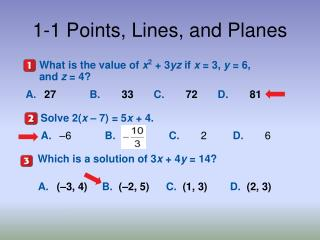DownloadDownload Presentation1-1 Points, Lines, and Planes

# 1-1 Points, Lines, and Planes

Download Presentation## 1-1 Points, Lines, and Planes

- - - - - - - - - - - - - - - - - - - - - - - - - - - E N D - - - - - - - - - - - - - - - - - - - - - - - - - - -
##### Presentation Transcript

1. A.–6 B.C.2 D.6 1-1 Points, Lines, and Planes What is the value ofx2 +3yz if x = 3, y = 6, and z = 4? A. 27 B. 33 C. 72 D. 81 Solve 2(x – 7) = 5x + 4. Which is a solution of 3x + 4y = 14? A. (–3, 4) B. (–2, 5) C. (1, 3) D. (2, 3) 5-Minute Check 3

2. Then/Now You used basic geometric concepts and properties to solve problems. • Identify and model points, lines, and planes. • Identify intersecting lines and planes.

3. Vocabulary • definition • defined term • space • undefined term • point • line • plane • collinear • coplanar • intersection

4. point • line • plane • collinear • coplanar • Intersection • space What do you think are basic geometry figures?

5. Answer: The line can be named as line a. There are three points on the line. Any two of the points can be used to name the line. Name Lines and Planes A. Use the figure to name a line containing point K.

6. Answer: The plane can be named as plane B. You can also use the letters of any three noncollinearpoints to name the plane.plane JKM plane KLM plane JLM Name Lines and Planes B. Use the figure to name a plane containing point L. The letters of each of these names can be reordered to create other acceptable names for this plane. For example, JKM can also be written as JMK, MKJ, KJM, KMJ, and MJK. There are 15 different three-letter names for this plane.

7. A.line X B.line c C.line Z D. A. Use the figure to name a line containing the point X.

8. B. Use the figure to name a plane containing point Z. A.plane XY B.plane c C.plane XQY D.plane P

9. A. Name the geometric shape modeled by a 10  12 patio. Answer: The patio models a plane. B. Name the geometric shape modeled by a button on a table. Answer: The button on the table models a point on a plane. A. Name the geometric shape modeled by a colored dot on a map used to mark the location of a city. A. Point B. line segment C. plane D. none of the above

10. Draw a surface to represent plane R and label it.

11. Draw a line anywhere on the plane.

12. Draw dots on the line for point A and B. Label the points.

13. Label the intersection point of the two lines as P.

15. Answer: There are an infinite number of points that are collinear with Q and R. In the graph, one such point is T(1, 0).

16. A. B.C.D. A. Choose the best diagram for the given relationship. Plane D contains line a, line m, and line t, with all three lines intersecting at point Z. Also, point F is on plane D and is not collinear with any of the three given lines.

17. A. B. C.D.

18. A. How many planes appear in this figure? Answer: There are two planes: plane S and plane ABC.

19. B. Name three points that are collinear. Answer: Points A, B, and D are collinear.

20. C. Are points A, B, C, and D coplanar? Explain. Answer: Points A, B, C, and D all lie in plane ABC, so they are coplanar.

21. Answer: The two lines intersect at point A.

22. A. How many planes appear in this figure? A. one B. two C. three D. four

23. B. Name three points that are collinear. A.B, O, and X B.X, O, and N C.R, O, and B D.A, X, and Z

24. C. Are points X, O, and R coplanar? A. yes B. no C. cannot be determined

25. A. point X B. point N C. point R D. point A

26. Assignment 1-1 p. 8. 13-21 all, 22-44 even